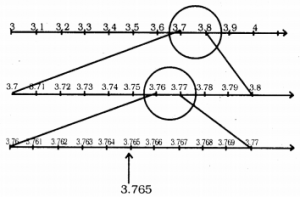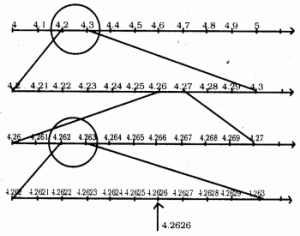# KSEEB Solutions for Class 9 Maths Chapter 1 Number Systems Ex 1.4

In this chapter, we provide KSEEB SSLC Class 9 Maths Chapter 1 Number Systems Ex 1.4 for English medium students, Which will very helpful for every student in their exams. Students can download the latest KSEEB SSLC Class 9 Maths Chapter 1 Number Systems Ex 1.4 pdf, free KSEEB SSLC Class 9 Maths Chapter 1 Number Systems Ex 1.4 pdf download. Now you will get step by step solution to each question.

## Karnataka Board Class 9 Maths Chapter 1 Number Systems Ex 1.4

Question 11.
Visualize 3.765 on the number line, using successive magnification.
Visualization of 3.765 on the number line:We know that 3.765 lies between 3 and 4. So, divide the portion between 3 and 4 into 10 equal parts and look at the portion between 3.7 and 3.8 through a magnifying glass. Then, 3.765 lies between 3.7 and 3.8 Fig. Now, we imagine dividing this again into 10 equal parts. The first mark will represent 3.71, the next 3.72, and so on. To see this clearly, we magnify this as shown in Fig. Then, 3.765 lies between 3.76 and 3.77 Fig. So, let us focus on this portion of the number line Fig and imagine to divide it again into 10 equal pans Fig. Here, we can visualize the 3.761 is the first mark, 3.762 is the second mark, and so on.

Question 2.
Visualise 4.26¯¯¯¯¯ on the number line, up to 4 decimal places.
Visulaisation of 4.26¯¯¯¯¯ upto 4 decimal places on number line :All Chapter KSEEB Solutions For Class 9 Maths

—————————————————————————–

All Subject KSEEB Solutions For Class 9

*************************************************

I think you got complete solutions for this chapter. If You have any queries regarding this chapter, please comment on the below section our subject teacher will answer you. We tried our best to give complete solutions so you got good marks in your exam.

If these solutions have helped you, you can also share kseebsolutionsfor.com to your friends.

Best of Luck!!